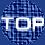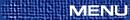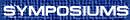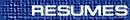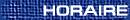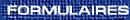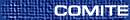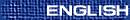Category Theory / Théorie des catégories
(R. Paré, Organizer)

MICHAEL BARR, McGill University, Montreal, Quebec  H3A 2K6
Absolute homology

Call two maps, f,g: C ® C¢, of chain complexes absolutely homologous if for any additive functor F, the induced Ff and Fg are homologous (induce the same map on homology). It is known that the identity is absolutely homologous to 0 iff it is homotopic to 0 and tempting to conjecture that f and g are absolutely homologous iff they are homotopic. This conjecture is false, but there is an equational characterization of absolute homology. I also characterize left absolute and right absolute (in which F is quantified over left or right exact functors).

RICK BLUTE, University of Ottawa, Ottawa
Constructing *-autonomous categories

We begin by considering a new example of a *-autonomous category due to T. Ehrhard, called the category of ``finiteness spaces''. This is at once a generalization of the category of sets and relations, and the category of coherence spaces. One essentially adds a notion of orthogonality to the usual category of sets and relations, and only considers morphisms respecting that orthogonality. The resulting category carries the full structure of a Seely model.

This idea of adding a notion of orthogonality to monoidal categories seems quite general, and should lead to a number of new models of linear logic. We consider several notions of such orthogonality, including one applied to a category of formal distributions, first defined by the author and P. Panangaden.

M. BUNGE, Department of Mathematics and Statistics, McGill University, Montréal, Québec  H3A 2K6
A Van Kampen theorem for Grothendieck toposes

This work is part of a larger program of developing some aspects of algebraic topology and functional analysis for Grothendieck toposes regarded as generalized topological spaces. The principal motivation for this particular paper was to provide an interpretation, in topos-theoretic terms, of (the calculation of) knot groups via branched coverings, as advocated by R. H. Fox (1957) in topology. To this end, we prove that the 2-category of Grothendieck toposes (by which we mean toposes bounded over a given base topos S), is extensive in an appropriate (bicategorical) sense, thus generalizing the well-known extensivity (A. Carboni, S. Lack and R. C. F. Walters, 1993) of the category of topological spaces. This is then coupled with a bicategorical version of a (Seifert-)Van Kampen theorem of R. Brown and G. Janelidze (1997) involving descent, that we first prove and then apply to various fibrations of quantities (intensive, extensive, and of mixed type) on Grothendieck toposes. In the case of the fibration of covering projections over a topos (or of locally constant objects in a topos), the passage to a Van Kampen theorem for group(oid)s, as in topology, is effected by means of the construction (M. Bunge 1991) of the (Grothendieck, or ``coverings'') fundamental groupoid of an unpointed locally connected topos bounded over an arbitrary base toposd S.

(This is joint work with Stephen Lack, University of Sydney, Australia.)

R. COCKETT, University of Calgary, Calgary, Alberta
Games as polarized categorical proof theory

Categories of games are usually employed as the basis for providing a semantics for a free category carrying a specified structure. They are particularly attractive in this regard as their maps are combinatoric objects. This means that, in simple cases at least, the inequality of maps can be concretely exhibited.

Of course, any category has a combinatoric nature when its maps have a concrete normal form. What is interesting is that often a game theory (with a concrete normal form) can be extracted from a standard proof theory (which does not have a nice normal form) by ``polarizing'' the propositions and forcing the proofs to be viewed as interactions between the two polarities.

The talk will discuss some examples of this and the polarized categorical semantics which results.

PIERRE DAMPHOUSSE, Universite Francois Rabelais, Faculte des sciences, Parc de Grandmont, 37200  Tours, France
Groupe des automorphismes des la categorie des G-ensembles / The automorphisms of the category of G-sets

Nous décrivons à équivalence naturelle près le groupe des automorphismes de la catégorie des G-ensembles (et des applications G-invariantes) pour n'importe que groupe donné G, et nous utilisons cette description pour obtenir une action naturelle de S3 sur les cartes cellulaires.

We describe the group of automorphisms (up to natural equivalence) of the category of G-sets (and G-invariant maps) for any given group G, and we use this description to describe a natural action of S3 on cellular decompositions of a surface.

R. DAWSON, St. Mary's University, Halifax, Nova Scotia  B3H 3C3

The well-known Span construction adds right adjoints to a category, freely except for the Beck condition. If right adjoints are added completely freely a different construction arises which we will call P2. A special case of this, for the category 2, was computed by Schanuel and Street in 1986. This talk presents recent work by Robert Pare, Dorette Pronk, and the speaker, in which the the general construction is studied.

P2(C) is a 2-category whose objects are those of C, whose arrows are zigzags of arrows of C, and whose 2-cells are represented by certain diagrams that we call ``fences''. Fences do not obey the middle-four property, but appropriate equivalence classes of them do; these equivalence classes are the actual 2-cells. Various basic properties will be presented; in particular, it is shown that the equivalence relation on fences may be undecidable.

J. DUSKIN, Department of Mathematics, S.U.N.Y. at Buffalo, New York  14260-2900, USA
Nerves of bicategories and Simpson-Tamsamani weak 2-categories

In [Duskin 2002] the author gave a detailed definition of a simplicial set Ner(B) which completely encoded the structure of a weak 2-category B in the commonly accepted sense of a bicategory as defined in [Bénabou 1967]. Ner(B) was a subcomplex of its 2-Coskeleton and was isomorphic to its 3-Coskeleton, what we called a 2-dimensional Postnikov complex, and satisfied certain very weak ``Kan-like'' horn filling properties provided that that certain 2- and 1-simplex faces which occurred in the horns were members of a subset of (abstractly) invertible 2- and 1-simplices. We proved there that those 2-dimensional Postnikov complexes in which (1)  all degenerate 2-simplices are invertible and in which (2) all horns (x2[( x12) || (¬ )]x1 [( x01) || (¬ )]x0) Î Ù21(X·) have an an invertible 2-simplex filler, are exactly the nerves of bicategories. (Those in which every 2 and 1-simplex is invertible are exactly the nerves of bigroupoids, i.e., bicategories in which every 2-cell is an isomorphism and every 1-cell is an equivalence).

In this short talk we will compare this result with the double simplicial set characterization theorem which is the specialization of the multi-simplicial approach to weak n-categories of [Simpson 1997] and [Tamsamani 1995] as described in [Leinster 2002]. In Ner(B), each of the sets of simplices forms the set of objects of a natural category structure for which the face and degeneracy maps become functorial and the 0-simplices become the objects of a discrete category. The corresponding canonical Segal maps all admit right (in our orientation) adjoint sections and become equivalences provided one restricts in each dimension to the full subcategories whose n-simplex objects all have isomorphisms as their 2-cell interiors. The resulting bisimplicial set is a weak 2-category in the sense of Simpson-Tamsamani. Conversely, given a bisimplicial set C· which is a weak 2-category in the sense of Simpson-Tamsamani, one can construct a 2-dimensional Postnikov complex in which the objects of the category C2 @ C1×C0 C1 form the set of invertible 2-simplices and makes it the nerve of a bicategory. Thus the two definitions are effectively equivalent. In contrast to what the author asserted in the introduction to [Duskin 2002], the Simpson-Tamsamani definition does not force the resulting bicategory to have all of its 2-cells isomorphisms. The assertion was the result of an mistaken interpretation the Simpson-Tamsamani definition.

## References

[Bénabou 1967]
J. Bénabou, Introduction to Bicategories. Reports of the Midwest Category Seminar, Lecture Notes in Math. 47(1967), 1-77.

[Duskin 2002]
J. Duskin, Simplicial Matrices and the Nerves of Weak n-Categories I: Nerves of Bicategories. Theory and Applications of Categories (10) 9(2002), 198-308.

[Leinster 2002]
Tom Leinster, A Survey of Definitions of n-Categories, Theory and Applications of Categories (1) 10(2002), 1-70.

[Simpson 1997]
Carlos Simpson, Effective generalized Seifert-Van Kampen: how to calculate WX. xxx.lanl.gov/q-alg/9710011 v2, 19 Oct 1997.

[Tamsamani 1995]
Z. Tamsamani, Sur des Notions de n-Catégorie et n-Groupoid Non-Strict via des Ensembles Multi-Simpliciaux. xxx.lanl.gov/alg-geom/95102006 v2. 15 Dec 1995.

P. FREYD, University of Pennsylvania, Philadelphia, Pennsylvania, USA
Algebraic real analysis

The co-algebraic definition of the real closed interval gives rise, somewhat paradoxically, to an algebraic definition: the theory of ``interval algebras'' is given by a constant, a pair of unary operations, a binary operation and a finite set of equations; every non-trivial interval algebra has a simple quotient; the full category of simple interval algebras has a terminator, to wit, the real closed interval. Since every model of any consistent extension of the algebraic theory of the closed interval will therefore have a map back to the closed interval a ``completeness theorem'' of remarkable power is obtained. (The von Neumann integral for any compact group, as an example, can be obtained as a consequence.) And the complete theory of all continuous self-maps of the closed interval can be given an entirely syntactical characterization. (That complete theory includes a good part of differential calculus.)

More results on branched covers in topos theory

The application of branched covers to braid group orderings in  has led to a continued investigation of branched covers in topos theory [1, 2]. Lawvere's topos distributions, pure geometric morphisms, and complete spread geometric morphisms provide a framework by which we may examine branched covers. One aspect that we consider is a comparison of the notions of pure and complete spread (or closure) relative to different base toposes. A fact about this comparison can be paraphrased as follows: an object of a locally connected topos E over another topos S is E-closed in its S-closure. We comment on some consequences of this fact.

## References


M. Bunge and S. Niefield, Exponentiability and single universes. J. Pure Appl. Algebra 148(2000), 217-250.


J. Funk, On branched covers in topos theory. Theory Appl. Categ. (1) 7(2000), 1-22.


, The Hurwitz action and braid group orderings. Theory Appl. Categ. (7) 9(2001), 121-150.

D. GARRAWAY, Colby College, Waterville, Maine, USA
Generalized supremum-enriched categries and their sheaves

In this talk we explore taxons enriched in the monoidal category of complete lattices and the associated lax-semifunctors and lax-transformations. We first show that for Q a sup-taxon the semicategory laxinf(Qco,rel) is equivalent to the semicategory of bimodules on Q An immediate corollary then is that the category of Q-valued sets is equivalent to the category map(taxinf(Qco,rel) ). From this it follows that for H, a complete Heyting algebra, the category of sheaves on H is equivalent to the category map(taxinf(Hco,rel)). Thus a sheaf on a complete Heyting algebra is a structure preserving semifunctor.

A. JOYAL, UQAM
Kan complexes and quasi-categories

A quasi-category is a simplicial set satisfying the restricted Kan conditions of Boardman and Vogt. A quasi-category X has an associated homotopy category hX. We show that X is a Kan complex iff hX is a groupoid. The result plays a an important role in the theory of quasi-categories. We apply it to study initial objects in a quasi-category. We introduce limits and colimits of diagrams in a quasi-category.

F. LINTON, Wesleyan University, Middletown, Connecticut  06459, U.S.A
On associativity for tensor products in varieties

One may introduce multilinear maps in the setting of any concrete category, and speak of tensor products of sequences of objects there as universal solutions to the problem of ``linearizing'' multilinear maps. Classical experience (abelian groups, modules over fixed commutative rings, pointed sets, etc.) leads to the expectation that iterated binary tensor products should associate, and therefore serve as sequential tensor products. For varieties that are entropic, by which is meant that each defining operation is a homomorphism in the variety, this expectation is indeed fulfilled. That impediments arise in general, though, is seen already in the variety of (associative) semigroups. There is, however, an astonishing plethora of examples of varieties in which, despite the failure of entropy, associativity nonetheless prevails. What little we know regarding the success or failure of associativity in absence of entropy will be shared here.

J. MACDONALD, University of British Columbia, Vancouver, British Columbia  V6T 1Z2
Embedding of algebras

We look at some general conditions for determining when the unit of an adjunction is monic when the generated monad is defined on a category of algebras. Among other possible applications these conditions will be shown to provide a common approach to some classic embedding theorems in algebra.

M. MAKKAI, McGill University, Montreal, Quebec  H3A 2K6
Comparing definitions of weak n-category for low values of n

The main topic is the comparison of the Gordon/Power/Street definition of tricategory with the multitopic 3-category (Hermida/Makkai/Power and Makkai) and with the 3-dimensional theta category (Joyal). The 2-dimensional case, which is well-understood, will be discussed for the purposes of introduction. Partial results in other dimensions will be mentioned.

S. NIEFIELD, Union College
Homotopy pullbacks, lax pullbacks, and exponentiability

We consider a unified approach to homotopy pullbacks, lax pullbacks, and pseudo-pullbacks via the notion of an E-pullback, where E is an object of a category E with finite limits. It turns out that E-pullbacks of objects over B exist in E if and only if the exponentials BE exist in E.

The goal of the talk is to discuss E-exponentiable morphisms of E, i.e., morphisms for which a certain E-pullback functor has a right adjoint. In particular, we will consider the relationship between E-exponentiability and ordinary exponentiability of morphisms of E, and apply these results to the categories of topological spaces, small categories, and Grothendieck toposes.

DORETTE PRONK, Department of Mathematics and Statistics, Dalhousie University, Halifax, Nova Scotia  B3H 3J5
Free extensions of double categories

The syntax of double categories is more complicated than the syntax for categories. Free extensions, being the ``polynomials'' of the double category, provide a valuable way of studying this. In this talk I discuss joint work with Robert Dawson and Robert Pare on free extensions of double categories by cells and arrows. I will give conditions under which there are normal forms for cells and arrows in the resulting double category. I will also show that if those conditions are not satisfied, equality for cells in the new double category may be undecidable. An application of this relating to the work by Brown and Mosa on symmetric double categories and connections will be discussed.

ROBERT ROSEBRUGH, Mount Allison
Updating views universally

A new treatment of view updates for the `sketch data model' (a semantic data model based on certain mixed sketches) will be presented. A database state is a model M of such a sketch E. Database systems require support for views, since view support allows application programs to continue to operate unchanged when the underlying system is modified. Fully supporting views depends on a solution to the view update problem: when can a view update be propagated to the full database? We define a view to be a sketch morphism K :V ® E and a view database N to be the result of substitution of a model M along K. We say that a view database is updatable if it enjoys a universal property for substitution along K. We explain why this property gives a complete and correct treatment of view updatability. and determines a solution to the view update problem. We also show that, in various contexts, updatability can be guaranteed independently of the view database.

This is joint work with Michael Johnson.

PHIL SCOTT, University of Ottawa, Ottawa, Ontario
Yoneda techniques and decision problems

The word problem for arrows in free cartesian and bicartesian closed categories is intimately related to decision problems in typed lambda calculi. Solving the latter involves intricate proof-theoretic techniques (related to so-called operational semantics in computer science). In the last decade, model-theoretic ``reduction-free normalization'' has been developed, which is both strikingly faster in computing normal forms and is not based on rewriting theory at all (Berger & Schwichtenberg, LICS 1991). Such normalization techniques have been increasingly studied by categorists, starting with Altenkirch, Hofmann, & Streicher (CTCS, 1995). I will report on some recent works (e.g. Cubric, Dybjer, Scott (MSCS, 1998), Altenkirch, Dybjer, Hofmann, Scott (LICS, 2001)) which use Yoneda and sheaf methods to solve decision problems for free (bi-)ccc's. The methods resemble Joyal-Gorden-Power-Street coherence techniques, and may be interpreted as a kind of ``reverse Lambek program'': instead of using proof theory to solve coherence problems (a la Lambek), we use techniques from categorical coherence theory to solve decision problems in proof theory. If time permits, we discuss applications to other logical systems and categorical frameworks for reduction-free normalization via generalized logical relations.

R. SEELY, Department of Mathematics, McGill University, Montreal, Quebec  H3A 2K6
Poly bicategories of modules

Recently, with the notions of ``poly bicategory'' and ``linear bicategory'', we generalized several bicategorical notions to a setting where there were two (implicit or explicit) ``horizontal'' compositions. Poly bicategories have poly 2-cells which may be regarded as arrows with strings of 1-cells as domains and as codomains; the representability of such arrows by ordinary arrows with single object domains and codomains gives the notion of linear bicategory. A good higher dimensional structure based on lax functors can be built over poly (and linear) bicategories, consisting of ``poly functors'', ``poly modules'' between them, and ``poly transformations'' between poly modules: poly functors between poly bicategories P and P¢ together with poly modules and their transformations form a module poly bicategory provided P is representable and closed in the sense that every 1-cell has both a left and a right adjoint (in the appropriate linear sense). Usually constructing module (bi)categories requires some completeness and cocompleteness; in the present setting we can identify such conditions as necessary for the representability of the module poly bicategory, rather than for its existence.

This is joint work with J. R. B. Cockett and J. Koslowski.

On completion of a poset in a topos

Let P be a poset in a topos E. The canonical topology on the object of left (right) ideals of P induces a subobject [^(P)](\checkP). If we let [`(P)] be the MacNielle completion of P, there is an embedding [`(P)]\hookrightarrow [^(P)] which has a left exact left adjoint. Moreover there is an isomorphism lim¬ W @ [^(P)]. There is also c:W\hookrightarrow[^(P)] in E, which may represent ``characteristic sheaves'' in [^(P)].

Just as

 E/X \rightleftarrows E(X, W)
is an adjunction induced by the truth value 1® W, where the set of fixed points of the adjunction is the full subcategory SubE(X) Ì E/X. If we replace P by the natural numbers object N, the projection ([^(N)])leq®[^(N)] will also induce an adjunction

 E/X \rightleftarrows E(X, ^N ).
The fixed points E ® X of this adjunction may be called ``finite filters''.

J. WICK PELLETIER, York University, 4700 Keele St., Toronto, Ontario  M3J 1P3
Spatiality of involutive quantales

This talk on based on joint work with C. J. Mulvey. We are concerned with finding a notion of spatiality of involutive quantales which when applied to the spectrum MaxA of a C*-algebra A captures our intuitive sense of that Gelfand quantale as a space. The approach taken in defining such a notion is motivated by that of Giles and Kummer in their article on non-commutative spaces [Indiana Univ. Math. J. 21(1971)]. We show that X is spatial if, and only if, it has enough points in the sense defined earlier in terms of algebraically irreducible representations [Mulvey, Pelletier, J. Pure Appl. Algebra 159(2001)]. A consequence is that a locale is spatial as an involutive unital quantale precisely when it yields the topology of a classical topological space.

R. WOOD, Dalhousie University, Halifax
2-categories of algebras for double dualization 2-monads

Double dualization with respect to W, the ordered set of truth values, provides a 2-monad on ord, the 2-category of ordered sets, whose 2-category of algebras is that of (constructively) completely distributive (CCD) lattices, functions which preserve all suprema and all infima, and inequalities. However, (-,W):ordop®ord is not monadic. We show that there is a specialization of a generalization of ordered sets for which homming into W is 2-monadic and for which the 2-category of algebras is the above 2-category of CCD lattices. Our methodology is quite general and applicable to similar double dualization monads on 2-categories of the form V-cat, for suitable monoidal V. If time permits, such examples will be examined.